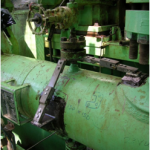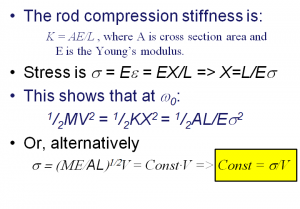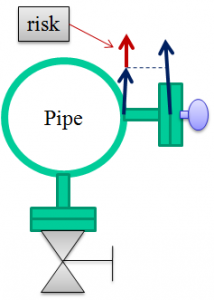# Small Bore – a Low Cost Element that can become very costlyPiping vibration can be troublesome in itself. Small Bore Fittings (a.k.a. Appurtenences) can be worse and usually are the things that first fatigue and break on a piping system and thereby add system risk.

There exists vibration fatigue risk criteria for piping vibration failure. These usually are expressed as vibration velocity for the simple reason that it is vibration velocity that is proportional to mechanical stress at resonance, i.e. at the a frequency where we expect vibration/stress to be the highest. Here is a small derivation as to why vibration velocity is proportional to mechanic stress at resonance.In short, we measure vibration velocity because it is easy, what we really want is mechanical stress.

Here is a link the most common vibration criteria in use for piping vibration. It relies on dynamic piping displacement and is derived from stress analysis of fundamental piping modes.

Reading the above reference you find that the vibration criteria is generic as it applies for the worst case of several fundamental piping vibration modes.

We would like to draw your attention to the small (bore) observation that a small bore, even though it rides on the pipe – it simply does not bend as is assumed for the main pipe. Yet, the piping vibration critera is applied also for Small Bore.

One motivation to this may be that if it is worse than the piping vibration – it adds risk for fatigue failure. In many cases, this is so but, it is far from a general truth. To exemplify why – imagine a case where piping vibration is 30 mm/s and small bore vibration is 20 mm/s.

• In this situation the relative vibration may be either 30 + 20 = 50 mm/s or 30-20 = 10 mm/s.

The former case, is worse than the latter because it involves a relative velocity of 50 mm/s for the small bore to accommodate, where the latter case only involves a relative velocity of 10 mm/s. Therefore, it is prudent to assess SBF risk using more than a single measurement channel.The examples list of why to handle small bore differently can be made much longer.

Qring believe that Small Bore vibration should be assessed different from piping vibration. In our opinion, best practice is